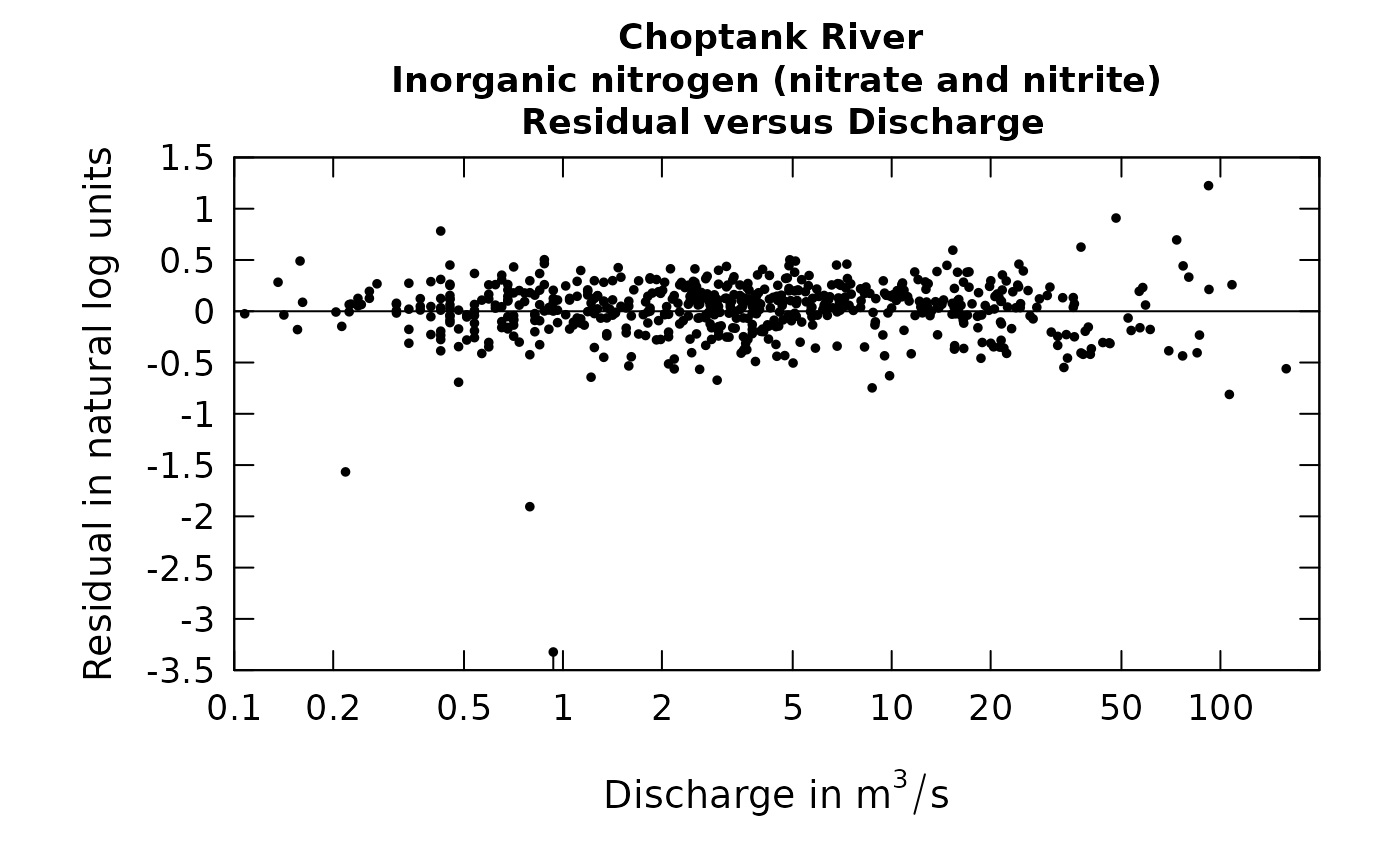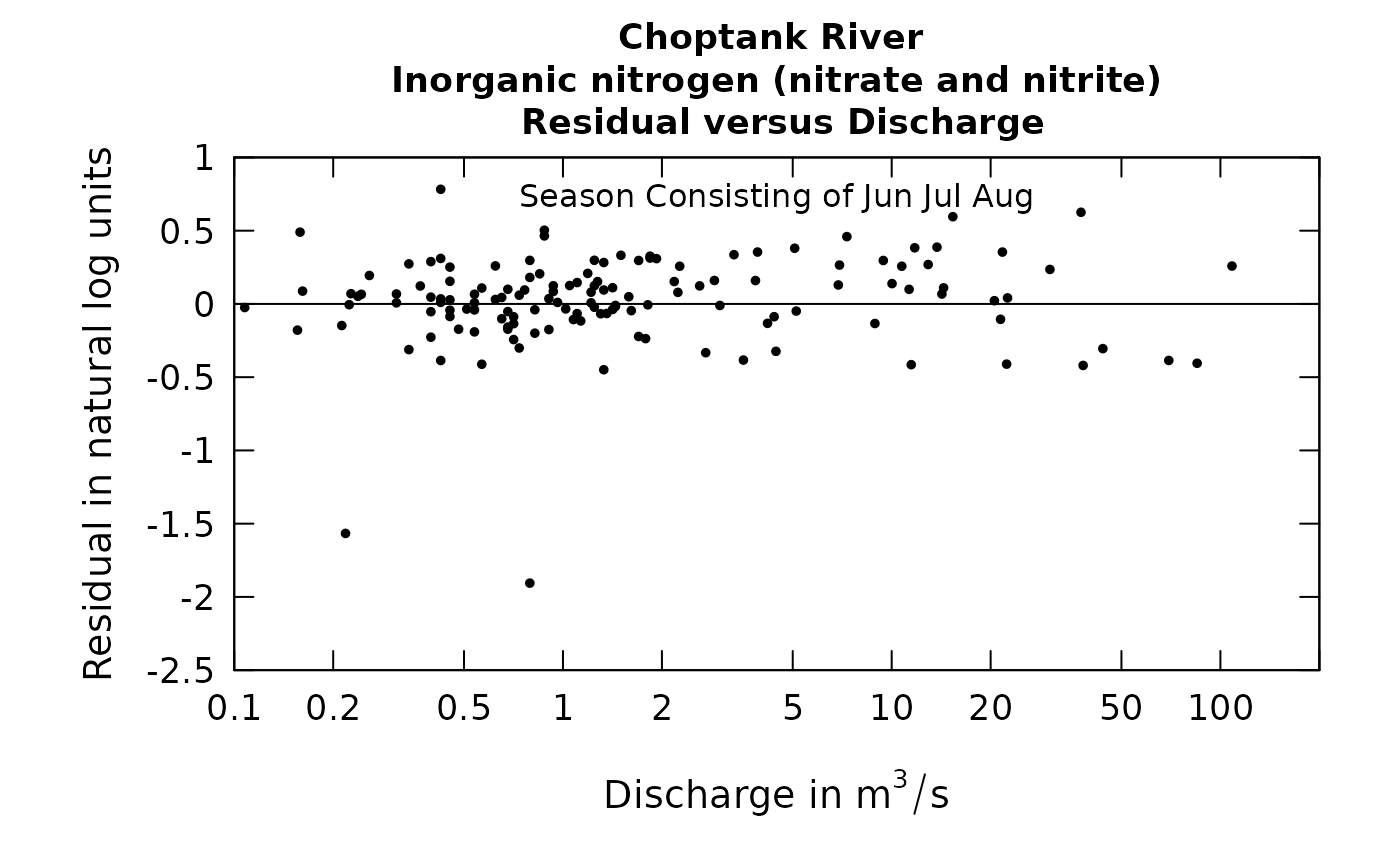This function produces a plot of the residuals from WRTDS, expressed in natural log concentration units versus the discharge shown on a log scale. The function also provides an alternative for viewing the standardized residuals, where the each residual is divided by its estimated standard error

Although there are a lot of optional arguments to this function, most are set to a logical default.

Data come from named list, which contains a Sample dataframe with the sample data, and an INFO dataframe with metadata.

## Usage

plotResidQ(eList, qUnit = 2, tinyPlot = FALSE, stdResid = FALSE,
printTitle = TRUE, col = "black", lwd = 1, cex = 0.8,
cex.axis = 1.1, cex.main = 1.1, rmSciX = FALSE, customPar = FALSE,
randomCensored = FALSE, usgsStyle = FALSE, ...)

## Arguments

eList

named list with at least the Sample and INFO dataframes

qUnit

object of qUnit class printqUnitCheatSheet, or numeric represented the short code, or character representing the descriptive name.

tinyPlot

logical variable, if TRUE plot is designed to be plotted small as part of a multipart figure, default is FALSE.

stdResid

logical variable, if TRUE it uses the standardized residual, if FALSE it uses the actual, default is FALSE

printTitle

logical variable if TRUE title is printed, if FALSE not printed (this is best for a multi-plot figure)

col

color of points on plot, see ?par 'Color Specification'

lwd

number line width

cex

numerical value giving the amount by which plotting symbols should be magnified

cex.axis

magnification to be used for axis annotation relative to the current setting of cex

cex.main

magnification to be used for main titles relative to the current setting of cex

rmSciX

logical defaults to FALSE, changes x label from scientific to fixed

customPar

logical defaults to FALSE. If TRUE, par() should be set by user before calling this function (for example, adjusting margins with par(mar=c(5,5,5,5))). If customPar FALSE, EGRET chooses the best margins depending on tinyPlot.

randomCensored

logical. Show censored residuals as randomized.

usgsStyle

logical option to use USGS style guidelines. Setting this option to TRUE does NOT guarantee USGS complience. It will only change automatically generated labels.

...

arbitrary graphical parameters that will be passed to genericEGRETDotPlot function (see ?par for options)

selectDays, genericEGRETDotPlot

## Examples

eList <- Choptank_eList
# Water year:
plotResidQ(eList)# Graphs consisting of Jun-Aug
eList <- setPA(eList, paStart=6,paLong=3)
plotResidQ(eList)